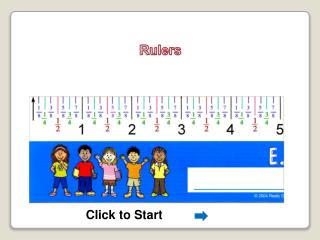DownloadDownload PresentationRulers

# Rulers

Télécharger la présentation## Rulers

- - - - - - - - - - - - - - - - - - - - - - - - - - - E N D - - - - - - - - - - - - - - - - - - - - - - - - - - -
##### Presentation Transcript

1. Rulers Click to Start

2. Rulers

3. Where is 0 ?

4. 0

5. 5 inches – 3 inches = 2 inches

6. Tall Mark Medium Mark Short Mark Inch mark (numbered)

7. Tall Mark

8. Tall Mark = Half Inch Mark

9. Tall Mark = ?

10. Tall Mark = ?

11. Measuring an object that ends on a Tall Half Inch Mark

12. How long is the pencil to the nearest quarter inch?

13. How long is the pencil to the nearest quarter inch?

14. How long is the pencil to the nearest quarter inch?

15. How long is the pencil to the nearest quarter inch?

16. How long is the pencil to the nearest quarter inch?

17. How long is the pencil to the nearest quarter inch?

18. How long is the pencil to the nearest quarter inch?

19. How long is the pencil to the nearest quarter inch?

20. When is a measurement to the nearest quarter inch?

21. When is a measurement to the nearest quarter inch?

22. When is a measurement to the nearest quarter inch?

23. When is a measurement to the nearest quarter inch?

24. Medium Mark

25. Medium Mark = Quarter Inch Mark

26. Medium Mark = ?

27. Medium Mark = ?

28. Medium Mark = ?

29. Medium Mark = ? 2 3 1

30. Medium Mark = ? 2 3 1 4

31. 1 2 4 4

32. 1 2 4 4 1 4 2 3

33. Measuring an object that ends on a Medium Quarter Inch Mark